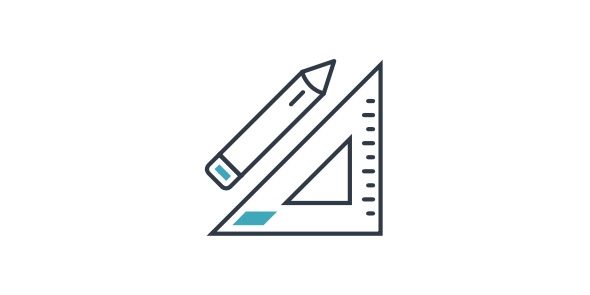# 7th Grade Test On Math! Practice Quiz! Trivia

74 Questions | Total Attempts: 296SettingsWhat we have here is a 7th grade test on math ideal for all the students who think of it as the hardest subject or can’t seem to understand how to solve some of the most basic mathematical problems. If you think you are up to the challenge this practice quiz is for you. Do give it a try and get refresh your understanding. All the best and keep practicing!

• 1.
What's 14 + 6?
• 2.
Round 41 to the nearest 10?
• 3.
What is 4 + 6?
• 4.
What's 16 + 2?
• 5.
What's missing? 200 + _______ = 1000 ?
• 6.
What's 5 + 8?
• 7.
What's 19 + 0 ?
• 8.
What's 8 + 7?
• 9.
What's 10 + 6?
• 10.
How many 10's in 90?
• 11.
What's 4 + 11?
• 12.
What fraction does this picture show?
• 13.
What's 6 + 13?
• 14.
What's 1 + 19?
• 15.
What is 6 + 11?
• 16.
What is 7 + 9?
• 17.
What is 2 + 18?
• 18.
What is 9 - 7?
• 19.
What is missing/ 8 + ______ = 10
• 20.
What is half of 20?
• 21.
What is 3 + 16?
• 22.
What is 6 + 9?
• 23.
Round 68 to the nearest ten?
• 24.
What is 5 - 3?
• 25.
What is 2 + 17?
Related TopicsBack to top